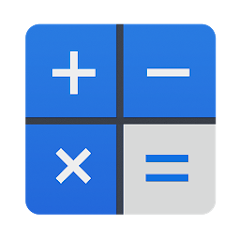# 모두의계산기 - 무료

50K+Everyone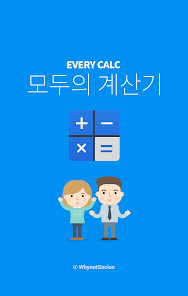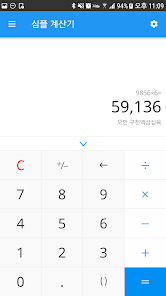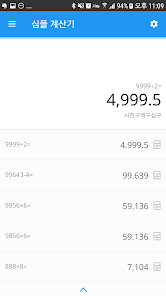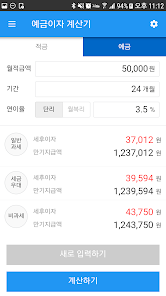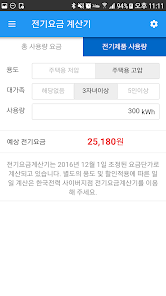Everyone's calculator helps you with a variety of calculations you need in your daily life.
From basic calculators to everyday life and finance, anyone can use it easily.
Swipe the screen on the left to bring up a list of useful calculators.
A cool and useful calculator is waiting for you!

※ The following calculators are available in the current version.
-Simple calculator
-Percent calculator
-Unit calculator
-Electricity rate calculator
-Deposit interest calculator
-Loan Interest Calculator
-Salary calculator
-Severance Pay Calculator
-Real Estate Fee Calculator
-Obesity calculator
-Abdominal obesity calculator
-Dutch Pay Calculator
-Yang/Lunar Calculator
-Tariff calculator
-Only age calculator
-Birth date calculator
-VAT calculator (total/supply price)
-D-day calculator

Updated on
Feb 16, 2021

## Data safety